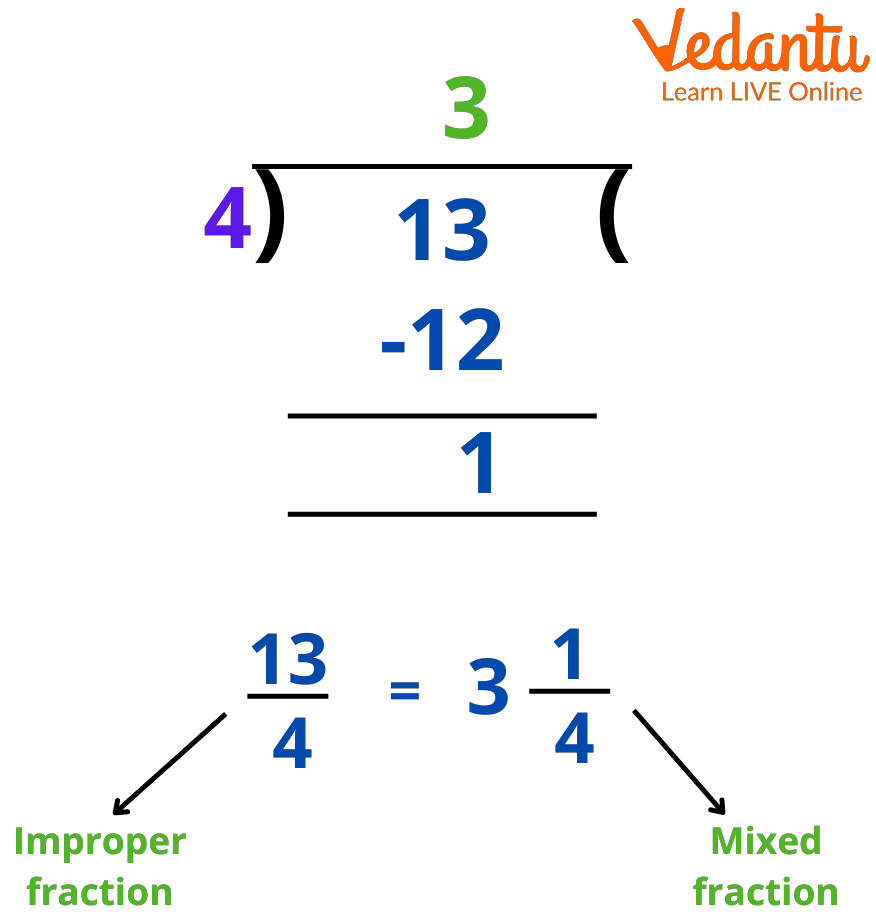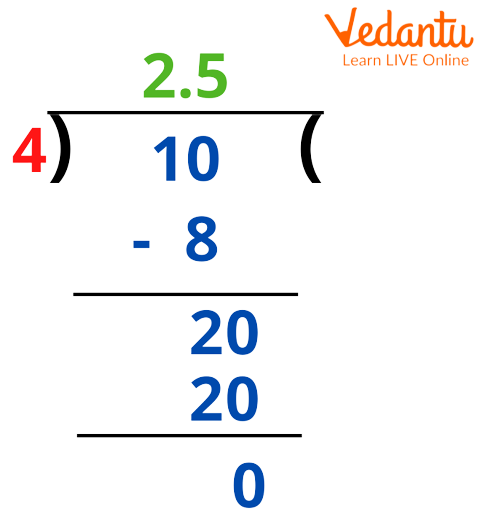Courses
Courses for Kids
Free study material
Offline Centres
More

# Improper Fractions - Definition, Conversion, and ExamplesLast updated date: 03rd Dec 2023
Total views: 160.2k
Views today: 3.60k## Introduction: Improper Fractions

Improper fractions are fractions having a numerator greater than the denominator. Here, fractions are representing something in parts. For example, you have 5 pieces of cake and after eating three pieces, you are left with two pieces. Now, how do you represent the remaining part? Well, it is simple, you write it as ⅖. Here, ⅖ is the fraction of the cake left with you.

In another case, you add 1 more piece of cake, then at present, you have$\frac{2}{5}$ + 1 = $\frac{7}{5}$ pieces of cake. So, here you notice that the numerator ‘7’ is greater than the denominator ‘5’, which means that this fraction is improper. We can also write $1\tfrac{2}{5}$ piece of cake. This is how we understand what an improper fraction is.

In real life, mixed fractions can be easily understood when compared to improper fractions. However, we can easily convert any improper fraction to a mixed number or vice-versa by following some basic steps that you will study in later sections on this page with solved examples.

## Concept of Improper Fractions

From the above text, we understand that improper fractions have numerators greater than or equal to its denominator. For example, $\frac{11}{5}$, $\frac{13}{4}$ are improper fractions.

Numerically, we find that these fractions are always equal to or greater than 1. We can write a mixed fraction from improper fractions. So, such a type of fraction carries a combination of a natural number and a proper fraction. The simplified form of an improper fraction becomes a mixed fraction, for example,$25\frac{4}{3}$ and $25\tfrac{4}{3}$ are mixed fractions. Numerically, we notice that a mixed fraction is always greater than 1. Also, we can rewrite a mixed fraction in the form of an improper fraction.

## Steps to Convert Improper Fractions to Mixed Numbers

Please note that the denominator of the mixed fraction form is always the same as that of the original fraction, i.e., of an improper fraction. Mixed numbers are the simplified form of improper fractions, that’s why it becomes important to learn this conversion. For converting an improper fraction to a mixed number, we need to follow the below-listed steps:

Step 1- Divide the numerator with the denominator, for example, if the fraction is $\frac{21}{4}$, then divide $\frac{21}{4}$

Step 2- Now, when you divide the numerator, you get a quotient and a remainder. Here, you get the quotient as 5 and the remainder as 1.

Step 3- Now, we arrange the values of the quotient, remainder, and divisor, i.e., 5, 1, and 4 in the following manner to express a fraction as a mixed fraction:

Quotient = $\frac{Remainder}{Divisor}$

Here, for the above values, you get $5\tfrac{1}{4}$ as a mixed fraction corresponding to the improper fraction of $\frac{21}{4}$

Like you can see under the division method for an improper fraction $\frac{13}{4}$:Converting Improper Fraction to a Mixed Fraction

## How To Solve Improper Fractions?

Solving an improper fraction is like solving any proper fraction, the only difference that comes here is, we have to simplify the answer and form mixed numbers.

Let's solve the improper fraction: 9/5 + 8/5.

Step 1: We notice that we have the same denominator for both the fractions. Therefore, we will directly add the numerators 9 and 8. We get 17. Thus, on adding improper fractions, we get $\frac{17}{5}$

Step 2: Simplifying the improper fraction (i.e., dividing 17 by 5), we will get 3 as a whole (which is a quotient), 2 as a numerator (remainder), and 5 as the denominator (divisor).

Using the formula Quotient = $\frac{Remainder}{Divisor}$

we get: $3\frac{2}{5}$, which is a mixed fraction corresponding to $\frac{17}{5}$

## Convert Improper Fraction to Decimal

Example 1: Convert 10/4 improper fraction to a decimal.

The first step we need to do is, divide 10 by 4. We know that 10 ÷ 4 = 2.5. Now, let us follow the long-division method here:Converting Improper Fraction to Decimal

Here, 10/4 is an improper fraction and 2.5 is a decimal. We can write 2.5 as 5/2, which is an improper fraction. Also, $2\tfrac{1}{2}$ makes it a mixed fraction because 2 is a whole number and $\frac{1}{2}$ is a proper fraction.

From the above text, we understand that solving improper fractions is similar to performing arithmetic operations on numbers simplifying the value of the answer so obtained. There are four arithmetric operators which are addition, subtraction, multiplication, and division.

## FAQs on Improper Fractions - Definition, Conversion, and Examples

1. What are proper and improper fractions?

Proper fractions are fractions that have a numerator lesser than the denominator, for example 2/3, 4/7, 8/19, and so on.

However, improper fractions are the opposite of proper fractions, in these fractions numerator ≥ denominator, for example, 9/5, 6/5, etc.

2. How to Subtract Improper Fractions?

We can subtract improper fractions by subtracting the values of their numerator and writing the common denominator as the denominator of the difference obtained. For this, we need to first take the LCM of the denominators to form a common denominator and subtract the numerators. After this, convert them into like fractions.